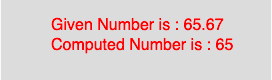# p5.js | floor() function

The floor() function in p5.js is used to calculate the floor value of a number. This function maps to the Math.floor() of javascript. It calculates the closest int value that is less than or equal to the value of the parameter.

Syntax

```floor(number)
```

Parameters: The function accepts only one parameter as mentioned above and described below:

• number : This parameter stores the number to compute.
• Below program illustrates the floor() function in p5.js:
Example:

 `function` `setup() { ` ` `  `    ``//create Canvas of size 270*80   ` `    ``createCanvas(270, 80); ` `} ` ` `  `function` `draw() { ` `    ``background(220); ` `    ``//initialize the parameter   ` `    ``let x = 65.67; ` `    ``//call to floor() function   ` `    ``let y = floor(x); ` `    ``textSize(16); ` `    ``fill(color(``'red'``)); ` `    ``text(``"Given Number is : "` `+ x, 50, 30); ` `    ``text(``"Computed Number is : "` `+ y, 50, 50); ` `} `

Output:Reference: https://p5js.org/reference/#/p5/floorMy Personal Notes arrow_drop_upCheck out this Author's contributed articles.

If you like GeeksforGeeks and would like to contribute, you can also write an article using contribute.geeksforgeeks.org or mail your article to contribute@geeksforgeeks.org. See your article appearing on the GeeksforGeeks main page and help other Geeks.

Please Improve this article if you find anything incorrect by clicking on the "Improve Article" button below.

Article Tags :

Be the First to upvote.

Please write to us at contribute@geeksforgeeks.org to report any issue with the above content.